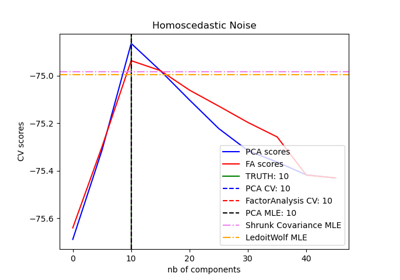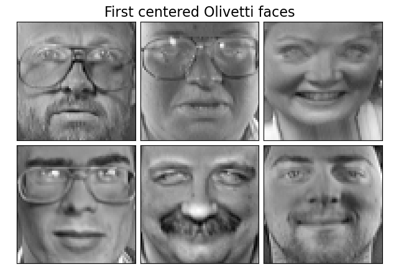# sklearn.decomposition.FactorAnalysis¶

class sklearn.decomposition.FactorAnalysis(n_components=None, *, tol=0.01, copy=True, max_iter=1000, noise_variance_init=None, svd_method='randomized', iterated_power=3, random_state=0)[source]

Factor Analysis (FA)

A simple linear generative model with Gaussian latent variables.

The observations are assumed to be caused by a linear transformation of lower dimensional latent factors and added Gaussian noise. Without loss of generality the factors are distributed according to a Gaussian with zero mean and unit covariance. The noise is also zero mean and has an arbitrary diagonal covariance matrix.

If we would restrict the model further, by assuming that the Gaussian noise is even isotropic (all diagonal entries are the same) we would obtain PPCA.

FactorAnalysis performs a maximum likelihood estimate of the so-called loading matrix, the transformation of the latent variables to the observed ones, using SVD based approach.

Read more in the User Guide.

New in version 0.13.

Parameters
n_componentsint | None

Dimensionality of latent space, the number of components of X that are obtained after transform. If None, n_components is set to the number of features.

tolfloat

Stopping tolerance for log-likelihood increase.

copybool

Whether to make a copy of X. If False, the input X gets overwritten during fitting.

max_iterint

Maximum number of iterations.

noise_variance_initNone | array, shape=(n_features,)

The initial guess of the noise variance for each feature. If None, it defaults to np.ones(n_features)

svd_method{‘lapack’, ‘randomized’}

Which SVD method to use. If ‘lapack’ use standard SVD from scipy.linalg, if ‘randomized’ use fast randomized_svd function. Defaults to ‘randomized’. For most applications ‘randomized’ will be sufficiently precise while providing significant speed gains. Accuracy can also be improved by setting higher values for iterated_power. If this is not sufficient, for maximum precision you should choose ‘lapack’.

iterated_powerint, optional

Number of iterations for the power method. 3 by default. Only used if svd_method equals ‘randomized’

random_stateint, RandomState instance, default=0

Only used when svd_method equals ‘randomized’. Pass an int for reproducible results across multiple function calls. See Glossary.

Attributes
components_array, [n_components, n_features]

Components with maximum variance.

loglike_list, [n_iterations]

The log likelihood at each iteration.

noise_variance_array, shape=(n_features,)

The estimated noise variance for each feature.

n_iter_int

Number of iterations run.

mean_array, shape (n_features,)

Per-feature empirical mean, estimated from the training set.

PCA

Principal component analysis is also a latent linear variable model which however assumes equal noise variance for each feature. This extra assumption makes probabilistic PCA faster as it can be computed in closed form.

FastICA

Independent component analysis, a latent variable model with non-Gaussian latent variables.

References

Examples

>>> from sklearn.datasets import load_digits
>>> from sklearn.decomposition import FactorAnalysis
>>> transformer = FactorAnalysis(n_components=7, random_state=0)
>>> X_transformed = transformer.fit_transform(X)
>>> X_transformed.shape
(1797, 7)


Methods

 fit(X[, y]) Fit the FactorAnalysis model to X using SVD based approach fit_transform(X[, y]) Fit to data, then transform it. Compute data covariance with the FactorAnalysis model. get_params([deep]) Get parameters for this estimator. Compute data precision matrix with the FactorAnalysis model. score(X[, y]) Compute the average log-likelihood of the samples Compute the log-likelihood of each sample set_params(**params) Set the parameters of this estimator. Apply dimensionality reduction to X using the model.
__init__(n_components=None, *, tol=0.01, copy=True, max_iter=1000, noise_variance_init=None, svd_method='randomized', iterated_power=3, random_state=0)[source]

Initialize self. See help(type(self)) for accurate signature.

fit(X, y=None)[source]

Fit the FactorAnalysis model to X using SVD based approach

Parameters
Xarray-like, shape (n_samples, n_features)

Training data.

yIgnored
Returns
self
fit_transform(X, y=None, **fit_params)[source]

Fit to data, then transform it.

Fits transformer to X and y with optional parameters fit_params and returns a transformed version of X.

Parameters
X{array-like, sparse matrix, dataframe} of shape (n_samples, n_features)
yndarray of shape (n_samples,), default=None

Target values.

**fit_paramsdict

Returns
X_newndarray array of shape (n_samples, n_features_new)

Transformed array.

get_covariance()[source]

Compute data covariance with the FactorAnalysis model.

cov = components_.T * components_ + diag(noise_variance)

Returns
covarray, shape (n_features, n_features)

Estimated covariance of data.

get_params(deep=True)[source]

Get parameters for this estimator.

Parameters
deepbool, default=True

If True, will return the parameters for this estimator and contained subobjects that are estimators.

Returns
paramsmapping of string to any

Parameter names mapped to their values.

get_precision()[source]

Compute data precision matrix with the FactorAnalysis model.

Returns
precisionarray, shape (n_features, n_features)

Estimated precision of data.

score(X, y=None)[source]

Compute the average log-likelihood of the samples

Parameters
Xarray, shape (n_samples, n_features)

The data

yIgnored
Returns
llfloat

Average log-likelihood of the samples under the current model

score_samples(X)[source]

Compute the log-likelihood of each sample

Parameters
Xarray, shape (n_samples, n_features)

The data

Returns
llarray, shape (n_samples,)

Log-likelihood of each sample under the current model

set_params(**params)[source]

Set the parameters of this estimator.

The method works on simple estimators as well as on nested objects (such as pipelines). The latter have parameters of the form <component>__<parameter> so that it’s possible to update each component of a nested object.

Parameters
**paramsdict

Estimator parameters.

Returns
selfobject

Estimator instance.

transform(X)[source]

Apply dimensionality reduction to X using the model.

Compute the expected mean of the latent variables. See Barber, 21.2.33 (or Bishop, 12.66).

Parameters
Xarray-like, shape (n_samples, n_features)

Training data.

Returns
X_newarray-like, shape (n_samples, n_components)

The latent variables of X.

## Examples using sklearn.decomposition.FactorAnalysis¶